# Shading Effect on Flow Rate of Solar DC Water Pump

Shading Effect on Flow Rate of Solar DC Water Pump
Department of Power Techniques Engineering, Faculty of Technical Engineering Collage, Northern Technical ‎University, Mosul 41001, Iraq

Corresponding Author Email:
Fawaz.sultan@ntu.edu.iq
Page:
309-314
|
DOI:
https://doi.org/10.18280/i2m.200603
29 October 2021
|
Accepted:
16 December 2021
|
Published:
31 December 2021
| Citation

OPEN ACCESS

Abstract:

Partial shading (PS) has a negative impact not only on the shaded Photo Voltaic PV modules/arrays but also on the power generated by the partially shaded photovoltaic (PSPV) system. It decreases the output power of the (PV) system and adds to hot fleck issues, which may result in the thermal breakdown of shaded PV modules. Solar water pumping is based on a (PV) system that converts solar energy into electrical energy to power a DC motor. This paper includes a practical investigation on the DC pump performance, as well as the influence of (1/4, 1/2, 3/4, and completely) shading on the performance of the DC pump motor. The current study shows that by Using maximum power point tracking (MPPT) control, the motor voltage will be stabilized and not affected by the increase in the shading area.

Keywords:

partial shading, PV system, pump performance, solar water

1. Introduction

Solar water pumps are water pumps that are driven by electricity that is generated from photovoltaic solar cells . Solar energy systems (PV) form a primary source of electricity generation that is deployed with diesel pumps in places where there is a lot of sunlight in order to avoid the high cost of operating diesel pumps. Solar systems are characterized by being scalable, with capacities of several watts for other uses, for example timekeeping systems, farm doors, and hundreds of kilowatts for homes and farms. It is possible to deploy a large number of solar systems in a finite number of sites instead of a single central system [2, 3].

Solar energy systems are best suited for the process of withdrawing groundwater and transferring it to large reservoirs, as it works without interruption throughout the day . The process of withdrawing groundwater at night is not correct for pumps that operate with solar cells, because this leads to an additional cost for the operation of the pump .

This article provides a practical assessment of the DC pump's performance, as well as the effect of shading (1/4, 1/2, 3/4, and totally) on the DC pump motor's performance. The current study shows that by using MPPT control, the motor voltage will be stabilised and not affected by the increase in the shading area. The novel aspect of this research is that partial shade has a detrimental effect on not just shading solar modules/arrays, but also on the amount of energy generated by the partially shading photovoltaic system. It reduces the (PV) system's output power and contributes to hot fleck concerns, which can result in the thermal breakdown of shaded PV modules.

2. Solar Water Pump Principle

PV technology is used in solar water pumping, which transforms sunlight into power to pump water. The PV panels are linked to a motor (DC or AC) that turns the electrical energy from the PV panels into mechanical energy, which the pump subsequently converts to hydraulic energy. Three primary aspects influence a solar pumping system's capacity to pump water: pressure, flow, and power to the pump [6-10].

For design purposes, pressure may be defined as the amount of effort expended by a pump to lift a certain amount of water to a storage tank. The elevation difference between the water source and the storage tank determines the amount of effort required by a pump. A specified quantity of power will be required by the water pump, which must be provided by a PV array. Figure 1 is an example of a direct-coupled DC solar photovoltaic water pumping system using MPPT .

## 1.png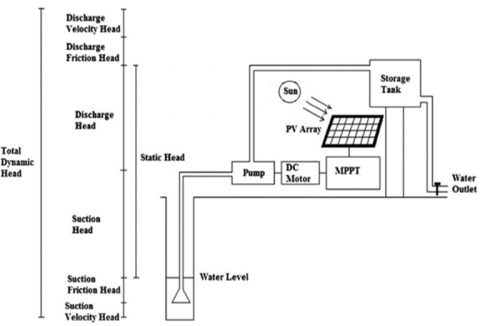Figure 1. Direct coupled solar photovoltaic water pumping system with MPPT

A direct coupled SPVWP is depicted in Figure 1. It consists of a photovoltaic array as the power source, a storage tank to store water for the system's 24 hour operation, a maximum power point tracking (MPPT) system to extract the maximum amount of energy from the photovoltaic array, and a DC surface mounted/submersible/floating motor pump set. The mounting structure for the photovoltaic array can be fixed or sun tracking, depending on the consumer need and cost. To operate the pump set during the day, night, and cloudy conditions, either a battery or a water tank can be utilised to store electricity or water. The pump set and other components of the SPVWP system must conform to applicable national and international standards.

3. Mathematical Model of PV Array

3.1 Characteristics under uniform conditions

The electrical model represents the solar cell with two diodes showing in Figure 2. Its current-voltage properties were calculated by the next equations [12-14]:

$\mathrm{I}=\mathrm{I}_{\mathrm{ph}}-\mathrm{I}_{\mathrm{d} 1}-\mathrm{I}_{\mathrm{d} 2}-\mathrm{I}_{\mathrm{sh}}$              (1)

The photo current is considered as in expression (2), the current diodes are considered as in the following expressions (3) and (4) consecutively:

## 2.png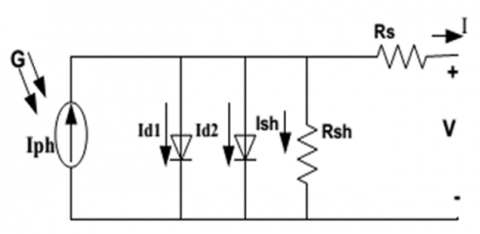Figure 2. Photovoltaic cell model

$\mathrm{Iph}=(\mathrm{Iph}, \mathrm{n}+\mathrm{KiDT}) \frac{\mathrm{G}}{\mathrm{Gn}}$             (2)

$\mathrm{Id} 1=\mathrm{I} 01\left[\exp \left(\frac{\mathrm{V}+\mathrm{IRs}}{\mathrm{a} 1 \mathrm{VT} 1}\right)-1\right]$               (3)

$\mathrm{Id} 2=\mathrm{I} 02\left[\exp \left(\frac{\mathrm{V}+\mathrm{IRs}}{\mathrm{a} 2 \mathrm{VT} 2}\right)-1\right]$             (4)

$\mathrm{I} 01=\mathrm{I} 02-\frac{\mathrm{Iscn}+\mathrm{KiDT}}{\exp \left[\mathrm{V} 0 \mathrm{cn}+\frac{\mathrm{KvDT}}{\mathrm{Vt}} \quad \right]-1}$              (5)

The shunt current and the panel voltage are determined in formulas (6) and (7) as:

$Ish =\frac{\mathrm{V}+\mathrm{RsI}}{\mathrm{Rsh}}$          (6)

$\mathrm{Vm}=\mathrm{Ns} * \mathrm{~V}$               (7)

3.2 Mathematical model of DC pump (LSQB SERIES DC MOTOR)

Many PV water pumping systems employ direct current (DC) motors rather than alternating current (AC) motors because they may be directly coupled to PV arrays, resulting in a reasonably simple system. Because of its higher starting torque, a permanent magnet DC (PMDC) motor is preferred in PV systems over other types of DC motors. The electrical model of a permanent magnet direct current (PMDC) motor is shown in Figure 3. When the motor turns, it produces a reverse emf, also known as a counter electromotive force, which is defined as an electric potential (E) proportional to the angular speed of the rotor. The armature circuit's DC voltage equation is ref.  obtained from the comparable circuit :

$V=I . R a+K \omega$              (8)

where, Ra is the armature resistance.

The back emf is E=K·w where: K is the constant, and w is the angular speed of rotor in rad/sec.

## 3.png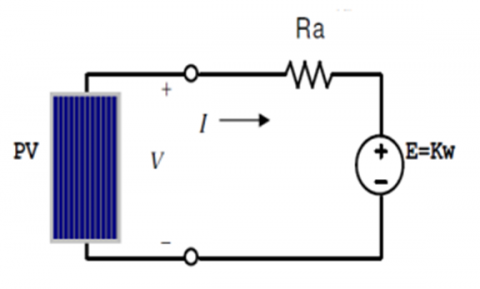Figure 3. Electrical model of PMDC motor

The motor shaft torque can be written as:

$T=T_{\varepsilon \mu}-T_{0}$             (9)

where, T denotes the motor shaft torque, $T_{\varepsilon \mu}$  the electromagnetic torque, and $T_{0}$ the torque, denoting the mechanical (friction and windage) and iron losses. The armature current is subjected to electromagnetic torque by:

$T=K_{T} I$               (10)

where, $K_{T}$  denotes the torque constant. At no-load, armature losses are negligible and may be ignored. Brush losses can also be ignored as a first-order approximation, resulting in the motor shaft output power P= Tω being represented as:

$P=K_{T}\left(I-I_{0}\right) \omega$                (11)

Using the knowledge that $K_{E}=K_{T}$, the constants for the above model are derived using the manufacturer's rated data and Io from a no-load test. Motor productivity may now be expressed in the following way:

$\eta_{\text {motor }}=K_{T} \frac{I-I_{0}}{I} \frac{\omega}{V}$           (12)

The applied voltage and rotating speed affect the current Io. A motor no load test can be used to determine these dependencies.

4. Methodology

The PV string, which consists of 20 PV modules, is utilized to test the behavior of this research. The open circuit voltage of the PV modules is 21.6 V, the short circuit current is 7.34 A, the ideality factor is 1.5, and the series resistance is 0 ohm. For all of the case studies, T = 25℃ is employed. Each PV module in the PV string is equipped with one bypass diode. As shown in Figure 4, there are four experiment configurations established utilizing the PV string: four modules shaded, eight modules shaded, twelve modules shaded, and sixteen modules shaded. Figure 5 displays the Flowchart of solar DC pump performance according to shading effect.

## 4.png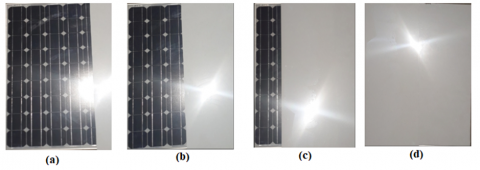Figure 4. The procedures of measurement the water flow rate and head according the DC pump model that used in this work

## 5.png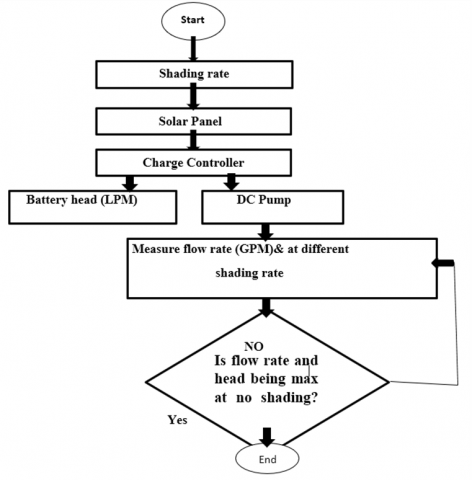Figure 5. Flowchart of solar DC pump performance according to shading effect

5. Experimental Part

The experimental part is implemented depending on the solar board as shown in Figure 6.

## 6.png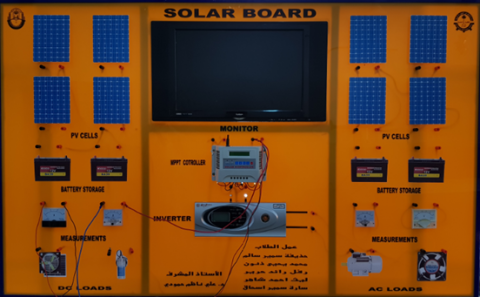Figure 6. Soler board employed in current study

The experimental board include:

1. PV panel

PV panel which used in this project is shown in Figure 7 and has specifications as given in Table 1.

## 7.png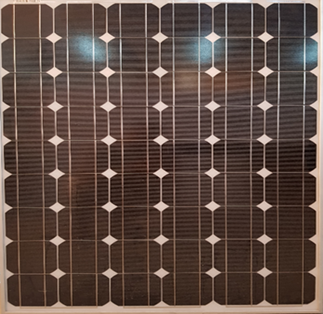Figure 7. PV panel

Table 1. Specifications of PV panel

 Related Maximum Power Pmax 120W Open-Circuit Voltage Voc 31.4V Short-Circuit Current Isc 5.05A Voltage at Pmax Vmp 26.0V Current at Pmax Imp 4.62A
1. MPPT controller

MPPT controller that used in this project is shown in Figure 8 and it has specifications as given in Table 2.

Table 2. Specifications of MPPT controller

 Rated Charge Current 50A Rated Discharge Current 50A Rated Voltage 12V/24V Auto Distinguish Over-Charge Protection 13.7/27.4V

## 8.png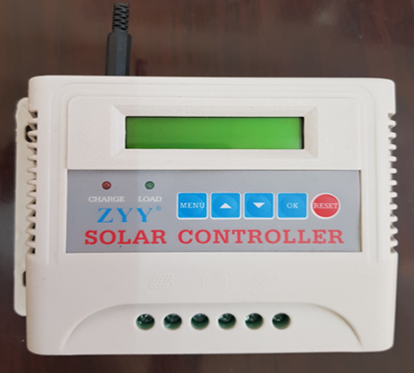Figure 8. MPPT controller

1. DC Pump specifications

DC pump that used in this work is shown in Figure 9 and has specifications as given in Table 3.

## 9.png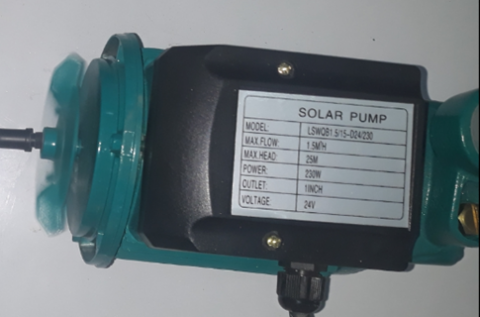Figure 9. DC pump

Table 3. Specifications of DC pump

 Model LSQB 1.5/15-D24/230 Max. Flow 1.5m3/h Max. Head 25m Power 230W Outlet 1inch Voltage 24V
6. Results and Discussion

In this section, DC pump performance as well as the influence of (1/4, 1/2, 3/4, and completely) shading on the performance of the DC pump motor have been analyzed and studied. Table 4 presents DC pump speed vs Vp panel voltage.

Table 4. DC pump speed vs Vp panel voltage

Figure 10 represents the relationship between PV panel voltage, pump speed with respect to shading rate in addition to the relation between PV panel voltage and pump speed during shadow stages.

## 10.png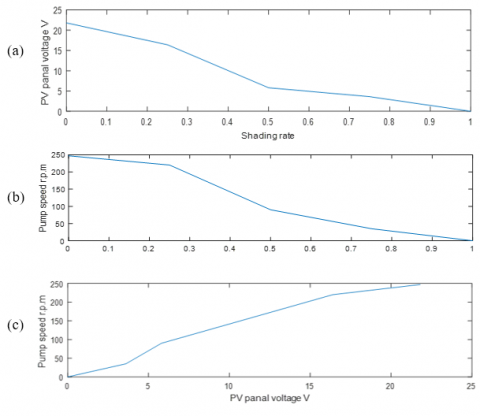Figure 10. (a) PV panel voltage vs shading rate, (b) PV panel voltage vs shading rate, and (c) DC pump speed vs shading rate

Table 5 represents the DC pump current values at different shading area without using MPPT controller.

Table 5. Current values at different shading area

Figure 11 shows are the current flow in DC motor is reduced at different insolation. the current flow is reduced from (1.11 A) to zero when become full shading.

## 11.pngFigure 12 shows are the DC pump voltage is remained constant (11.5V) due to using MPPT controller. But the speed of DC pump at different insolation is reduce from 250 r.p.m to 130 r.p.m when become full shading area. Table 6 presents DC pump voltage vs its speed by using MPPT controller.

Figure 13 shows are the current flow in DC motor is reduced at different insolation. the current flow is reduced from (1.1 A) to (0.36A) when become full shading area were using MPPT controller. Table 7 shows the values of DC pump current with respect to the shading area under using MPPT controller.

Flow rate and Head: The values of flow rate and the head of the water after connected the DC pump directly to the PV panel are shown in Table 8.

## 12.png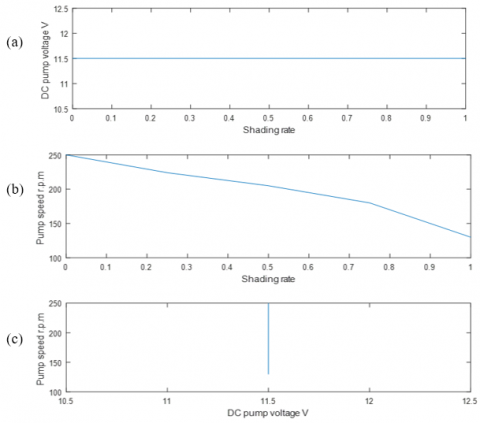Figure 12. (a) PV panel voltage vs shading rate, (b) DC pump speed vs shading rate, and (c) DC pump speed vs PV panel voltage

Table 6. DC pump voltage vs its speed by using MPPT controller

 Shading DC voltage (motor voltage) Speed (r.p.m) No. shad 11.5 V 250 1/4 11.5 V 224 1/2 11.5 V 205 2/3 11.5 V 180 Full 11.5 V 130

## 13.png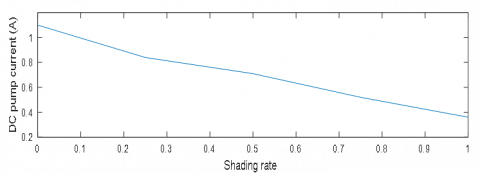Figure 13. The relationship between the DC pump current against the shading rate of the PV cell

Table 7. The values of DC pump current with respect to the shading area under using MPPT controller

From above Figure 14, the values of water flow rate and water head are reduced as the shading area is increased this due to reducing the PV panel output power as respect to sunlight beclouded, where the rate flow and the head of water before operated the DC pump are 2.5 and 9.6 this case is similar to that obtained from full shading because of full shading the DC pump is stopped.

Table 8. Values of DC pump flow rate with respect to the shading area under using MPPT controller

## 14.png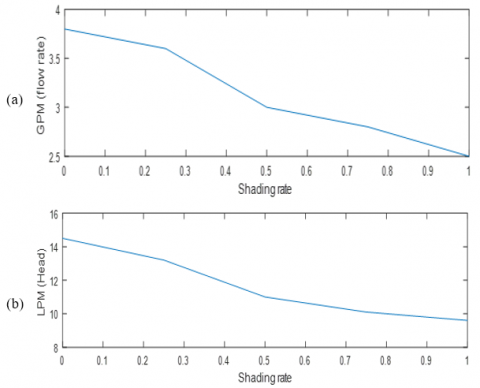Figure 14. DC pump rate of water flow against the water head

7. Conclusions

This article provides a practical analysis of the DC pump's performance, as well as an examination of the effect of shade on the DC pump motor's performance at 1/4, 1/2, 3/4, and entirely. The current study reveals that when the solar cells are not shaded, the panel voltage is as high as possible, and thus the pump speed is as high as feasible. And when we increase the shading area from 1/4 to 1/2 until the solar cells are totally shaded, the solar panel voltage drops to zero, and the pump speed drops to zero as the pump current drops to zero, rendering the solar cells unusable. Furthermore, by using MPPT control, the motor voltage is stabilised at 11.5 and is unaffected by the increased shade area, while the DC pump current and speed are decreased.

References

 Eker, B. (2005). Solar powered water pumping systems. Trakia Journal of Sciences, 3(7): 7-11. http://www.uni-sz.bg.

 Lakra, A., Gupta, K., Murmu, K., Prasad, D., Nath, V. (2019). Study and development of solar-powered water pumping system. In Proceedings of the Third International Conference on Microelectronics, Computing and Communication Systems, pp. 655-660. Springer, Singapore. https://doi.org/10.1007/978-981-13-7091-5_56

 Andrada Gascón, P., Castro, J. (2007). Solar photovoltaic water pumping system using a new linear actuator. Renewable Energy and Power Quality Journal, 2007(5): 321-1-321-6. http://hdl.handle.net/2117/14422.

 Foster, R., Cota, A. (2014). Solar water pumping advances and comparative economics. Energy Procedia, 57: 1431-1436. https://doi.org/10.1016/j.egypro.2014.10.134

 Setiawan, A.A., Purwanto, D.H., Pamuji, D.S., Huda, N. (2014). Development of a solar water pumping system in Karsts Rural Area Tepus, Gunungkidul through student community services. Energy Procedia, 47: 7-14. https://doi.org/10.1016/j.egypro.2014.01.190

 Pardo, M.Á., Cobacho, R., Bañón, L. (2020). Standalone photovoltaic direct pumping in urban water pressurized networks with energy storage in tanks or batteries. Sustainability, 12(2): 738. https://doi.org/10.3390/su12020738

 Shehadeh, N.H. (2015). Solar-powered pumping in Lebanon - A comprehensive guide on solar water pumping solutions. https://reliefweb.int/report/world/solar-powered-pumping-lebanon-comprehensive-guide-solar-water-pumping-solutions.

 Mohanty, A., Ray, P.K., Viswavandya, M., Mohanty, S., Mohanty, P.P. (2018). Experimental analysis of a standalone solar photo voltaic cell for improved power quality. Optik, 171: 876-885. https://doi.org/10.1016/j.ijleo.2018.06.139

 Pardo, M.Á., Manzano, J., Valdes-Abellan, J., Cobacho, R. (2019). Standalone direct pumping photovoltaic system or energy storage in batteries for supplying irrigation networks. Cost analysis. Science of the Total Environment, 673: 821-830. https://doi.org/10.1016/j.scitotenv.2019.04.050

 Pardo, M.A., Riquelme, A., Melgarejo, J. (2019). A tool for calculating energy audits in water pressurized networks. AIMS Environ. Sci., 6(2): 94-108. https://doi.org/ 10.3934/environsci.2019.2.94

 Chandel, S.S., Naik, M.N., Chandel, R. (2015). Review of solar photovoltaic water pumping system technology for irrigation and community drinking water supplies. Renewable and Sustainable Energy Reviews, 49: 1084-1099. https://doi.org/10.1016/j.rser.2015.04.083

 Shannan, N.M.A.A., Yahaya, N.Z., Singh, B. (2013). Single-diode model and two-diode model of PV modules: A comparison. In 2013 IEEE International Conference on Control System, Computing and Engineering, pp. 210-214. https://doi.org/10.1109/ICCSCE.2013.6719960

 Yilmaz, S., Yilmaz, A., Gunes, M., Ozcalik, H. (2013). Two-diode model performance analysis of photovoltaic panels. International Journal of Engineering Trends and Technology, 7: 2890-2895.

 Eltamaly, A.M., Farh, H.M. (2020). PV characteristics, performance and modelling. In Modern Maximum Power Point Tracking Techniques for Photovoltaic Energy Systems, Springer, Cham, 31-63. https://doi.org/10.1007/978-3-030-05578-3_2

 Naresh, B., Madhu, P., Prasad, K.R.K. (2011). Analysis of DC solar water pump and generalized photovoltaic model using Matlab/Simulink. UACEE International Journal of Advancements in Electronics and Electrical Engineering, 1(1): 19-24.